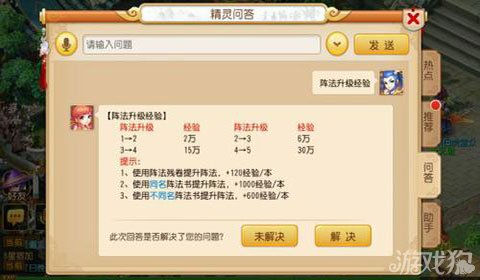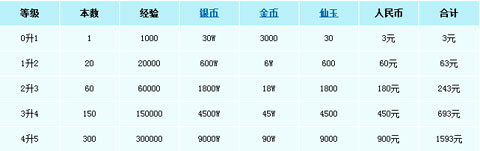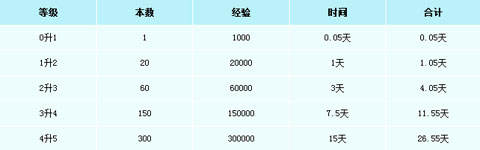### 梦幻西游手游满级阵法需要多久与银币消耗分享

2015-09-17 19:15:50(1000+20000+60000+150000+300000)/[(1*1000*20)+(9*600*20)]=531000/128000≈5天

1人赞过• A
• B
• C
• D
• F
• G
• H
• J
• K
• L
• M
• N
• P
• Q
• R
• S
• T
• W
• X
• Y
• Z